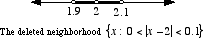# deleted neighborhood

Deleted Neighborhood

The proper name for a set such as {x: 0 < |xa| < δ}. Deleted neighborhoods are encountered in the study of limits. It is the set of all numbers less than δ units away from a, omitting the number a itself.

Using interval notation the set {x: 0 < |xa| < δ} would be (a – δ, a) ∪ (a, a + δ). In general, a deleted neighborhood of a is any set (c, a) ∪ (a, d) where c < a < d.

For example, one deleted neighborhood of 2 is the set {x: 0 < |x – 2| < 0.1}, which is the same as (1.9, 2) ∪ (2, 2.1).